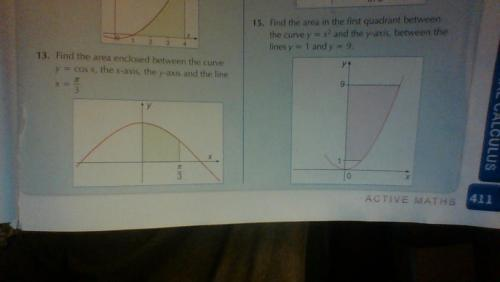## Recommended Posts

can some one help me with question 15. i am integrating it and trying this 1/3 (9)^3 -(1/3 (1)^3) and this gives me a answer of 242.66667 but the answer at the back of the book is 17 2/3##### Share on other sites

1. Always take a stab at an answer to get a rough idea. The area shaded is clearly all included in the area bounded by x=3 and y =9 that means it must be less than 27 square units

2. Your answer looks as if you are actually finding out the area under the curve y=x^2 between x = 1 and x=9. That is not what the question asks - can you see now what you are meant to do?

## Create an account

Register a new account